## Monday, November 30, 2015

### Individual Elements of Standard & Normalized CDF(2, 2): Conceptual Note 3 on Ripples in Mathematics

Introduction

This is my conceptual note 3 on Chapters 3 and 4 in "Ripples in Mathematics" by A. Jensen & A. la Cour-Harbo. This note continues to discuss the notation for CDF(2, 2) I introduced in conceptual note 2. I changed CDF(2, 2) to CDF(S, 2, 2, L, J) where S denotes the signal, which is a sequence of samples whose length is an integral power of 2. Specifically, the length of S is 2^J. L is the current scale or iteration. It starts at 0, which denotes the original, unmodified signal, and goes up to J. In this note, I will separate standard CDF(2, 2) and normalized CDF(2, 2). The normalization formulas are given in Ch. 3 in equations 3.40 and 3.41 on p. 23, where the signal samples, i.e., the elements of S, are normalized by sqrt(2) and the elements of D are normalized by 1/sqrt(2). I will also state the formulas for computing individuals elements of S and D at a given scale.

Changing CDF(S, 2,2, L, J) to SCDF(S,2,2,L,J) and NCDF(S, 2, 2, L, J)

Let us call CDF(S, 2, 2, L, J) standard CDF(2, 2) and change the notation to SCDF(S, 2, 2, L, J) where the first letter in the abbreviation stands for standard. The meaning of the other symbols in SCDF(S, 2, 2, L, J) is the same as in CDF(S, 2, 2, L, J). Let us also introduce the notation NCDF(S, 2, 2, L, J) to denote normalized CDF(2, 2). Figures 1 and 2 show how two scales of SCDF(S, 2, 2, L, J) and NCDF(S, 2, 2, L, J) are computed, respectively. I will still keep the notation CDF(S, 2, 2, L, J) to denote either SCDF(S, 2, 2, L, J) or NCDF(S, 2, 2, L, J) when the context permits safe omission of normalization.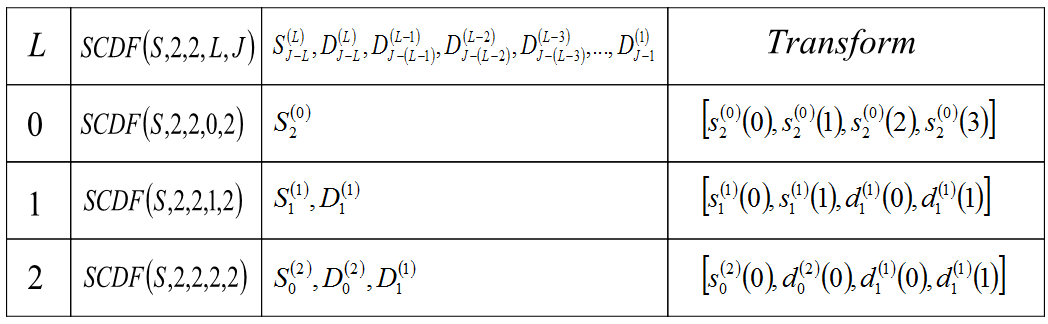Figure 1. Two scales of SCDF(S, 2, 2, L, J)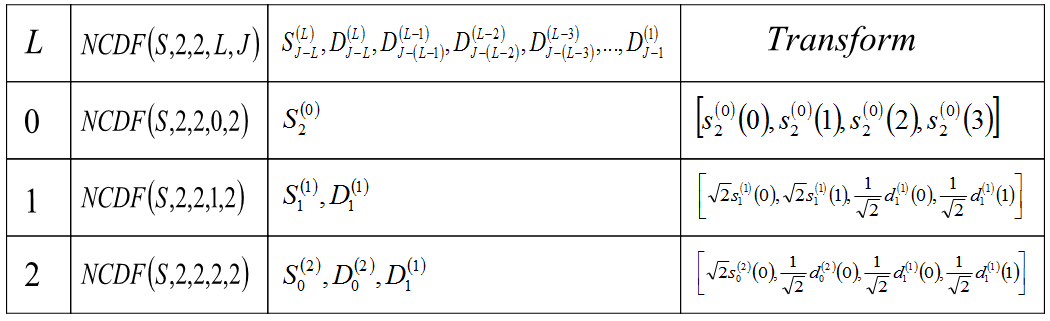Figure 2. Two scales of NCDF(S, 2, 2, L, J)

Formulas for Individual Elements at Two Scales of CDF(S, 2, 2, 2, 2)

Figures 3 and 4 give formulas for computing individual elements of CDF(S, 2, 2, 2, 2). Specifically, Figure 3 shows how to compute individual elements of CDF(S, 2, 2, 1, 2) and Figure 4 shows how to compute individual elements of CDF(S, 2, 2, 2, 2). If normalization is needed, then each element should be normalized, as shown in Figure 2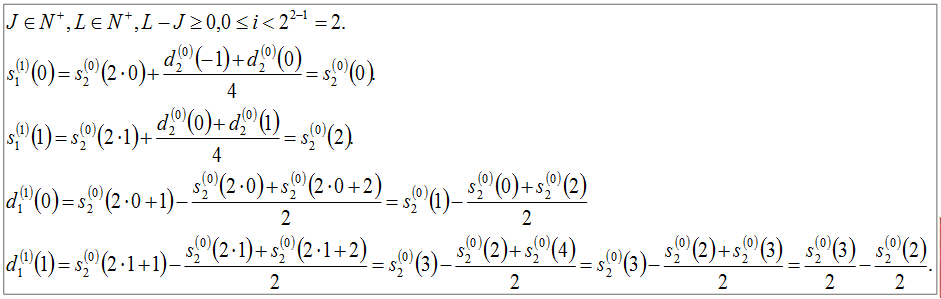Figure 3. Individual elements of CDF(S, 2, 2, 1, 2)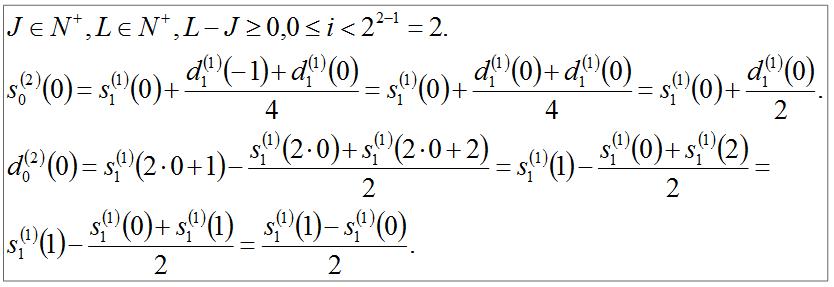Figure 4. Individual elements of CDF(S, 2, 2, 2, 2)

SCDF(S, 2, 2, 2, 2) Example

Figures 5 and 6 give two scales of SCDF(S, 2, 2, 1, 2) where the signal S = [1, 2, 3, 4].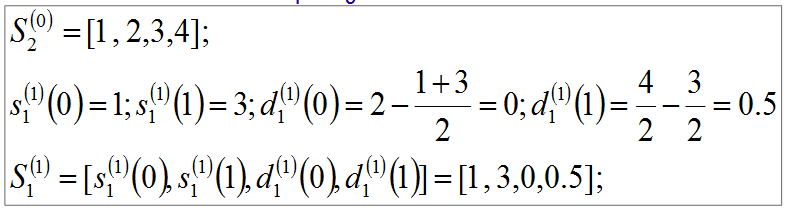Figure 5. SCDF(S, 2, 2, 1, 2)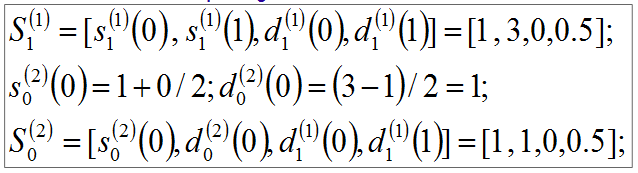Figure 6. SCDF(S, 2, 2, 2, 2)

NCDF(S, 2, 2, 2, 2) Example

Figures 7 and 8 give two scales of NCDF(S, 2, 2, 1, 2) where the signal S = [1, 2, 3, 4].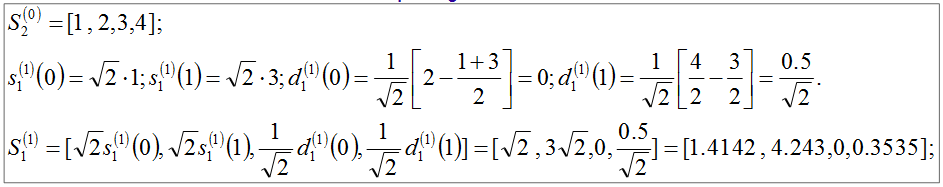Figure 7. NCDF(S, 2, 2, 1, 2)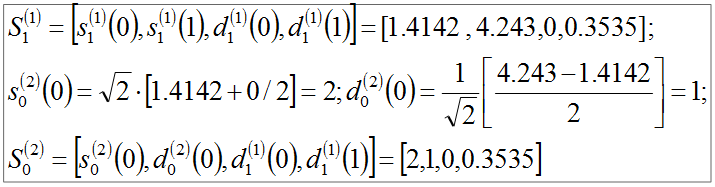Figure 8. NCDF(S, 2, 2, 2, 2)